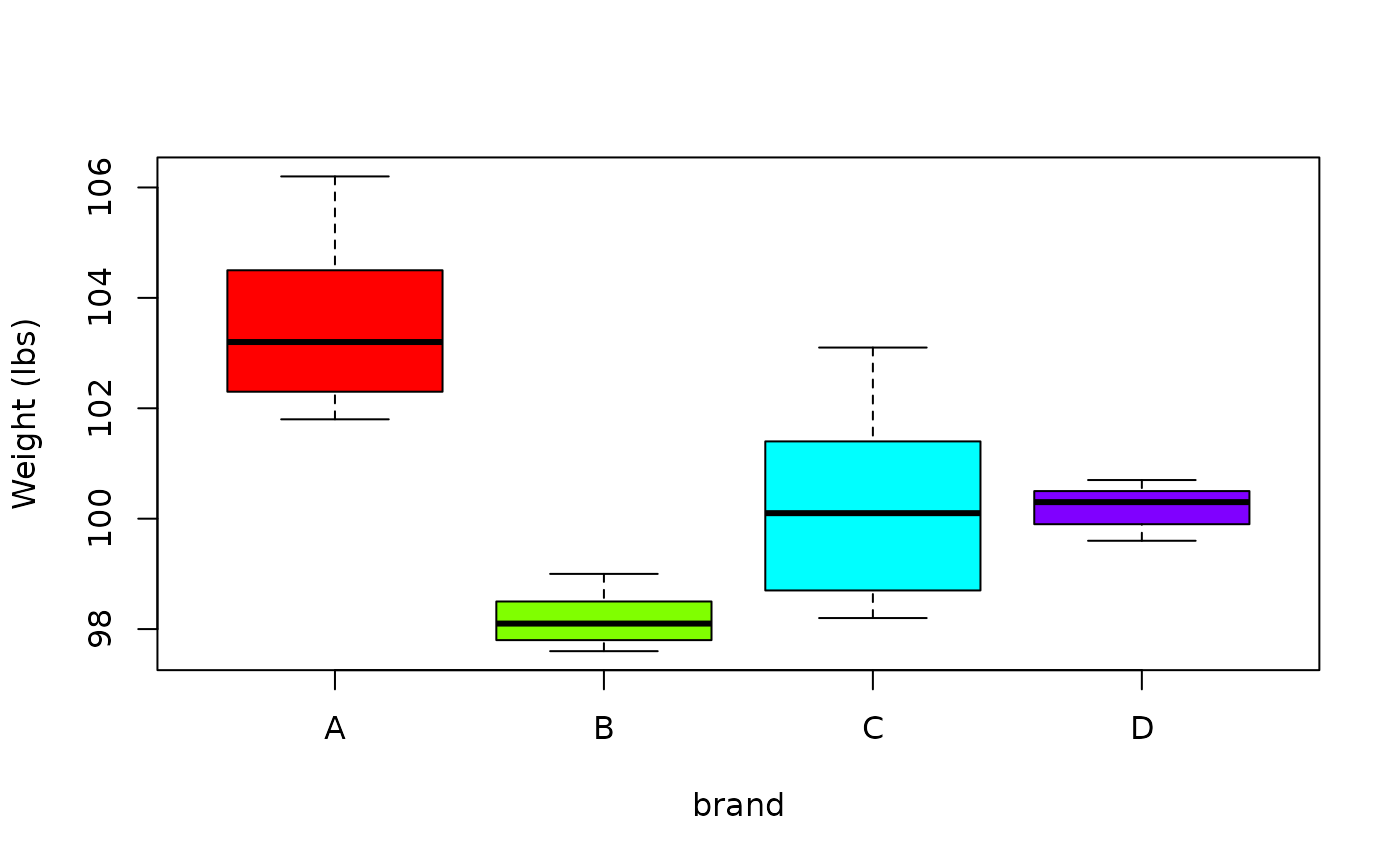Data for Exercise 1.89

Scales

## Format

A data frame/tibble with 20 observations on two variables

brand

variable indicating brand of bathroom scale (A, B, C, or D)

recorded value (in pounds) of a 100 pound weight

## Examples


boxplot(reading ~ brand, data = Scales, col = rainbow(4),
ylab = "Weight (lbs)")if (FALSE) {
library(ggplot2)
ggplot2::ggplot(data = Scales, aes(x = brand, y = reading, fill = brand)) +
geom_boxplot() +
labs(y = "weight (lbs)") +
theme_bw() +
theme(legend.position = "none")
}